# AP Psychology : Interpreting Results

## Example Questions

← Previous 1 3

### Example Question #1 : Interpreting Results

What kind of statistical test would be used to determine if there is a linear relationship between two variables?

T-test

Pearson correlation

ANOVA

Chi-square

Average

Pearson correlation

Explanation:

A Pearson correlation between two variables indicates that as one variable increases, another variable either increases or decreases by some amount in a linear manner.

### Example Question #2 : Interpreting Results

A study finds a strong negative correlation between the amount of chocolate a child consumes and the number of absences the child has from school. Which of the following values could express that correlation?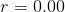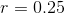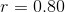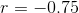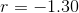Explanation:

Correlations range betweenand, withsignifying the strongest possible negative correlation between two variables,signifying the strongest possible positive correlation, andsignifying the complete absence of any correlation between two variables.

### Example Question #3 : Interpreting Results

What is an illusory correlation?

A perfect correlation between two variables

A type of Pearson product moment correlation commonly used in the field of social psychology

When one variable negatively predicts a second variable

The perception of a relationship where none exists

An illusion of a correlation (often called sans-stats)

The perception of a relationship where none exists

Explanation:

An illusory correlation occurs when someone thinks that two variables are related, but they are actually not (they have a correlation of 0).

### Example Question #1 : Interpreting Results

Which of these represents the strongest correlation coefficient?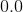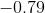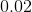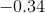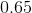Explanation:

Correlation coefficients range from -1 to 1, with the strongest correlations being closer to -1 or 1. A correlation of 0 indicates no relationship between two variables. Negative correlations can be as strong or stronger than positive correlations; the most important factor is the magnitude of the correlation.

### Example Question #5 : Interpreting Results

Which term refers to the middle term in a distribution of data?

The mode

The mean

The range

The standard deviation

The median

The median

Explanation:

The median is the middle number in a data set. For example, the median of {3, 7, 4, 5, 8} is 5. The terms must first be placed in increasing order: {3, 4, 5, 7, 8}. From here, it becomes evident that 5 is the middle term, or median.

### Example Question #1 : Interpreting Results

Which of the following is an appropriate definition for statistics?

None of these

The negative or positive correlational relationship between different percentages

A subjective interpretation of numerical data

Numerical data that shows the cause of a certain phenomenon

An analysis of numerical data

An analysis of numerical data

Explanation:

Statistics play a big role in much of the research in the field of psychology. In studying groups of people and individuals, their behaviors, reactions, etc. a researcher will have lots of numerical data on their hands. Statistics is the analysis of this numerical data.

### Example Question #7 : Interpreting Results

Carol did a study examining the link between male students' test scores and their alcohol consumption. Other researchers replicated her study in a similar setting and got back similar results, but argued that her study answers a different question than it was designed to answer.

Carol's study is __________.

Reliable, but not valid

Reliable and valid

None of the other answers is correct

Neither valid nor reliable

Valid, but not reliable

Reliable, but not valid

Explanation:

A study's validity is its ability to scientifically measure the concepts it was designed to measure. A study's reliability is its ability to yield similar results when replicated in a similar setting.

Because Carol's study could be replicated but does not answer the question it was designed to answer her study is reliable, but not valid.

### Example Question #1 : Interpreting Results

In a correlation experiment which number shows the weakest correlation?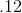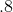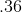Explanation:

The number describing two variables relationship is the correlation coefficient. This could show a positive correlation (+ number) or a negative correlation (- number). The further from 0 the coefficent is the stronger the correlation.

### Example Question #9 : Interpreting Results

When measuring central tendency, which of the following choices is known to be biased depending on the data set?

Median

Standard deviation

Mean

Percent error

Standard deviation of the mode

Mean

Explanation:

The mean is the average of a data set. On the other hand, the median is considered to be the 50th percentile—that is cutting the entire data set in half and representing the exact middle of the data set. The mean may be skewed depending on the data set. For instance, the median may be 50 but because of a few higher numbers, the mean could be 56. Using the mean as a measure of central tendency may cause the distribution to be a bit lopsided. Percent error is a statistical measure of the marginal error of actual results compared to expected results; therefore, percent error would not be the correct answer. Additionally, standard deviation is the measurement of the deviation from a group as a whole. This calculation is often conducted using averages (means); thus, the options standard deviation and standard deviation of the mode would also be incorrect.

### Example Question #1 : Interpreting Results

A researcher has determined through an experiment that moderate doses of ginseng have no effect on verbal memory; thus, he fails to reject the null hypothesis. In reality, the researcher missed a critical data point in his analysis that should have pointed towards a positive effect of ginseng on memory. This is an example of which of the following types of research error?

Lurking variables

Confounding variables

Type I error

Experimental drift

Type II error